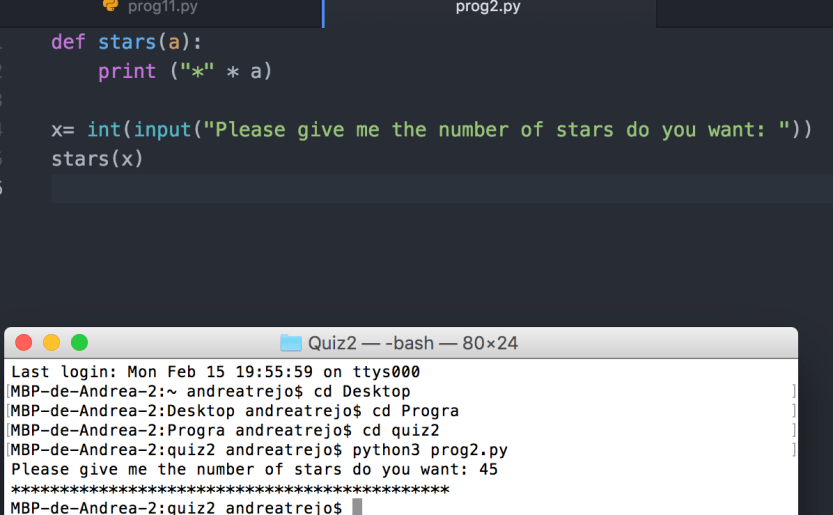And one or 2 more post for today, here’s the code for the 2 problems

Here’s the first part, in atom and the terminal

First I used 2 inputs and then defined the superpower which will allow us to generate the operation is this right here (x,y)

then the result will be z which is (x**y) and it will return it has the superpower!!!I had some problems with the second problem, but thanks to this blog I figured out how to do the solution in the easiest way possible! Thank you esenombredeusua 🙂

First we define a function that will allow us to print as many stars as we want in the same line def stars(a): then we multiply the string so it would be so much easier, I know this thanks to the pal aboveAnd last but not least, my code on Github

Quiz2